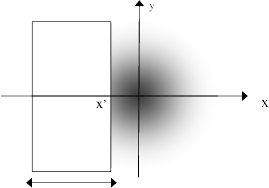# Comparison to Razor Blade Method¶

Scott Prahl

Mar 2021

In the past, translating a razor blade across a laser beam was the standard method for measuring a beam diameter. The total power would be measured for each position of the razor blade as it occluded the beam. The beam diameter could be found from a plot of the power versus position. See, for example,

Khosrofian and Garetz, “Measurement of a Gaussian laser beam diameter through the direct inversion of knife-edge data”, *Applied Optics*, **22**, 3406-3410, (1983).

In this notebook, we compare the results from lbs.beam_size() with that from a fit to a simulated razor blade experiment done on the same image.

If laserbeamsize is not installed, uncomment the following cell (i.e., delete the initial #) and execute it with shift-enter. Afterwards, you may need to restart the kernel/runtime before the module will import successfully.

:

#!pip install --user laserbeamsize

:

import imageio
import scipy.special
import numpy as np
import matplotlib.pyplot as plt

try:
import laserbeamsize as lbs

except ModuleNotFoundError:
print('laserbeamsize is not installed. To install, uncomment and run the cell above.')
print('Once installation is successful, rerun this cell again.')

pixel_size_mm = 3.75/1000           # pixel size in mm for the camera
repo = "https://github.com/scottprahl/laserbeamsize/raw/master/docs/"


## The fundamental mode TEM$$_{00}$$¶

The lowest order Hermite polynomials, $$H_0(x)=1$$ (and generalized Laguerre polynomials $$L_0^0(x)=1$$) are simply constants, so the fundamental ($$m=0, n=0$$) mode is a Gaussian. The electric field $$\mathcal{E}$$ is

$\mathcal{E}_{00}(x,y,z) \propto e^{−(x^2+y^2)/w^2} e^{−ik(x^2+y^2)/2R(z)} e^{i(\omega t−kz+\phi)}$

The irradiance $$E_{00}(x,y,z)$$ at a given point in space is defined as the power per unit area in a plane perpendicular to the direction of propagation. It is proportional to the square of the electric field

$E_{00}(x,y,z)\propto \mathcal{E}_{00}\cdot \mathcal{E}_{00}^* = E_{00} e^{−2(x^2+y^2)/w^2}$

and the proportionality constant $$E_{00}$$ can be expressed in terms of the total power $$P_{00}$$ of the beam by requiring the integral of the irradiance over $$x$$ and $$y$$ to equal $$P_{00}$$

$E_{00} = {2P_0\over\pi w^2}$If the beam is partially blocked (e.g., with a razor blade that stops all light with $$x>x'$$), then the power reaching the detector is

$P(x')=E_{00}\int_{-\infty}^{x'} e^{−2x^2/w^2}dx \int_{-\infty}^\infty e^{−2y^2/w^2}dy$

and the normalized power reaching the detector is

$\frac{P(x')}{P}=\frac{1}{2}\left[1+\mathrm{erf}\left(\frac{\sqrt{2}(x'-x_0)}{w}\right)\right]$

where $$\mathrm{erf}()$$ denotes the error function. The beam radius $$w$$ is found by fitting to the above expression.

### Sample Data¶

:

beam=imageio.imread(repo + 't-hene.pgm')

ym,xm = beam.shape
plt.imshow(beam,extent=[0,xm*pixel_size_mm,ym*pixel_size_mm,0],cmap='gray')
plt.show()### Simulated Horizontal Razor Blade Movement¶

Just use the image to figure out what the measured power would be for each position of the blade.

:

v,h = beam.shape
xval = pixel_size_mm * np.arange(0,h,1)
s = beam.sum(axis=0).cumsum()/beam.sum()
plt.scatter(xval[::30],s[::30], s=2)

#fit parameters found by trial and error
hw=0.8
hx0=2.45

fit = 0.5*(1+scipy.special.erf((-hx0+xval)*np.sqrt(2)/hw))
plt.plot(xval,fit)
plt.xlabel("Horizontal (mm)")
plt.ylabel("Cumulative Total")
plt.title("Simulated Razor Experiment (Horizontal)")
plt.xlim(0,5)
plt.ylim(-0.1,1.1)
plt.show():

#uses values from previous cell
z = beam[int(v/2),:]
plt.scatter(xval[::30],z[::30],s=2)

peak=190
fit = peak*np.exp(-2*(xval-hx0)**2/hw**2)
plt.plot(xval,fit)

plt.plot([hx0,hx0],[0,peak],':k')

plt.xlabel("Horizontal (mm)")
plt.ylabel("Gray Level")
plt.title("Horizontal Cross Section Through Beam")
plt.xlim(0,5)
plt.ylim(0,200)

ge2 = peak*np.exp(-2)
plt.annotate('w',xy=(hx0+hw/3,ge2+5))
plt.annotate('',xy=(hx0,ge2),xytext=(hx0+hw,ge2),arrowprops=dict(arrowstyle="<-"))
plt.show()### Simulated Vertical Razor Blade Movement¶

Same as before, but move vertically.

:

v,h = beam.shape
yval = pixel_size_mm * np.arange(0,v,1)
s = beam.sum(axis=1).cumsum()/beam.sum()
plt.scatter(yval[::30],s[::30], s=2)

vw=0.8
vx0=1.85

fit = 0.5*(1+scipy.special.erf((-vx0+xval)*np.sqrt(2)/vw))
plt.plot(xval,fit,'b')
plt.xlabel("Vertical (mm)")
plt.ylabel("Cumulative Total")
plt.title("Simulated Razor Experiment (Vertical)")
plt.xlim(0,3.5)
plt.ylim(-0.1,1.1)
plt.show():

#uses values from previous cell
z = beam[:,int(h/2)]
plt.scatter(yval[::30],z[::30],s=2)

peak=190
fit = peak*np.exp(-2*(yval-vx0)**2/vw**2)
plt.plot(yval,fit)

plt.plot([vx0,vx0],[0,peak],':k')

plt.xlabel("Horizontal (mm)")
plt.ylabel("Gray Level")
plt.title("Horizontal Cross Section Through Beam")
plt.xlim(0,5)
plt.ylim(0,200)

ge2 = peak*np.exp(-2)
plt.annotate('w',xy=(vx0+vw/3,ge2+5))
plt.annotate('',xy=(vx0,ge2),xytext=(vx0+vw,ge2),arrowprops=dict(arrowstyle="<-"))
plt.show()## Beam Radius based on slope¶

Another method to based on the derivative of the cumulative power. If the maximum value of this derivative is $$\alpha$$ then the beam radius is

$\alpha =\sqrt{\frac{2}{\pi}} \frac{1}{w}$

The advantage here is that not fitting is required.

:

v,h = beam.shape
xval = pixel_size_mm * np.arange(0,h,1)
s = beam.sum(axis=0).cumsum()/beam.sum()

# this takes the derivative of the cumulative sum with respect to the real position
alpha = xderiv.max()
w = 1/alpha*np.sqrt(2/np.pi)

plt.scatter(xval[::30],s[::30], s=2,color='red')
plt.scatter(xval[::20],xderiv[::20],s=2,color='blue')

plt.xlabel("Horizontal Position (mm)")
plt.ylabel("")
plt.title("Simulated Razor Experiment (Horizontal)")
plt.annotate("Derivative",xy=(3,0.5),color='blue')
plt.annotate("Cum. Sum",xy=(4,0.95),color='red')
plt.annotate(r"$\alpha$=%.2f"%alpha, xy=(1.5,0.95), color='blue')
plt.annotate("w=%.2f"%w, xy=(1.5,0.85), color='black')

plt.xlim(0,5)
plt.ylim(-0.05,1.05)
plt.show()## lbs.beam_size() comparison¶

The results are not exactly the same, but close. The centers match perfectly. The razor blade technique tends to return slightly larger beam radii than the lbs method.

:

xc,yc,dx,dy,phi=lbs.beam_size(beam)
lbs_xc = xc*pixel_size_mm
lbs_yc = yc*pixel_size_mm

lbs_wx = dx*pixel_size_mm/2
lbs_wy = dy*pixel_size_mm/2

print("value   razor   lbs")
print("        [mm]    [mm]")

print("  xc    %.2f    %.2f"%(hx0,lbs_xc))
print("  yc    %.2f    %.2f"%(vx0,lbs_yc))
print()
print("  wx    %.2f    %.2f"%(hw,lbs_wx))
print("  yx    %.2f    %.2f"%(vw,lbs_wy))

value   razor   lbs
[mm]    [mm]
xc    2.45    2.44
yc    1.85    1.84

wx    0.80    0.63
yx    0.80    0.61

[ ]: